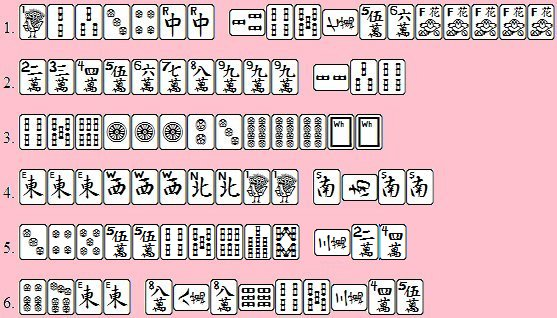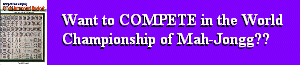Please click here if you do not see a Nav Frame at left and Header Frame above - you'll need them!

WEEKLY MAH-JONG

By Tom Sloper (湯姆)

April 16, The Year Of The Dog
Column #262

Chinese Official Tournament Rules. Scoring exercises. For each of the following waiting hands, how many points would you expect to earn? Assume win by discard, not the last tile of the wall, for each of these. Answers in italics at the bottom. For maximum enjoyment, don't check the answers until you've thought it through completely! If you see something I've missed (and you probably will), email me and we'll discuss on the bulletin board.1. 5D produces only Mixed Double Chow (1). The flowers are useless, of course, until the hand itself has met the minimum 8-point requirement. But 2D produces Chicken Hand (8).

2. If winning tile is 1C: Pure Straight, All Chows, One Voided Suit (16+2+1=19). But 4C or 7C only give you All Chows, Short Straight, One Voided Suit, Mixed Double Chows (2+1+1+1=5). 2C makes Short Straight, Terminal Pung, No Honors, and One Voided (1+1+1+1=4). 8C makes Short Straight, Terminal Pung, No Honors, Mixed Double Chows, and One Voided (1+1+1+1+1=5). 4C makes All Chows, One Voided (2+1=3). 7C makes All Chows, Short Straight, One Voided (2+1+1=4). 5C makes Terminal Pung, Mixed Double Chows, and One Voided only (1+1+1=3). The only way to win without 1C is with the very last tile in the wall. Shouldn't have melded.

3. If winning tile is 1D: Terminal Pung, Reversible Tiles, Two Concealed Pungs, Concealed Hand, Tile Hog (1+8+2+2+2=15). If 4D, drop Tile Hog (1+8+2+2=13). If winning tile is Wh: Dragon Pung, Reversible Tiles, Concealed Hand (2+8+2=12).

4. If winning tile is 1B: Little Four Winds, All Terminals & Honors, Half Flush, Two Concealed Pungs, Melded Kong (64+32+6+2+1=105). And if your seat is anything but N, add 2; if the round is anything but N, add 2. But if winning tile is N: Big Four Winds, Half Flush, All Terminals & Honors, Two Concealed Pungs, Melded Kong (88+6+32+2+1 =129). (Formerly controversial but now resolved; see column 259).

5. 3B nets All Chows, All Simples, Short Straight, Mixed Double Chows (2+2+1+1=6). But 6B earns Mixed Shifted Chows, All Chows, All Simples (6+2+2=10). 9B gets Mixed Shifted Chows, Short Straight, All Chows (6+1+2=9).

6. 5D produces only Mixed Shifted Chows (6). If winning tile is 8D: Chicken Hand (8).

Edited thanks to Andrew Feist, Tina Christensen, and "ithinc," sharp-eyed readers who saw things I missed.

Click the entries in the header frame, above, to read other columns.